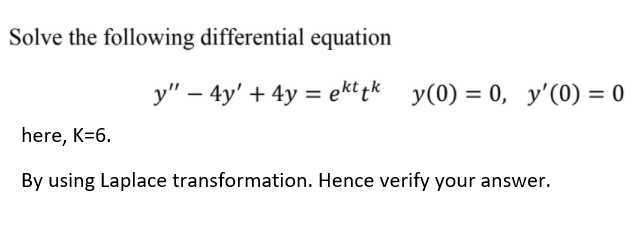### Create an Account

Already have account?

### Forgot Your Password ?

Home / Questions / Solve the following differential equation y" – 4y' + 4y = ekt tk y(0) = 0, y'(0) = 0 here,...

# Solve the following differential equation y" – 4y' + 4y = ekt tk y(0) = 0, y'(0) = 0 here, K=6. By using Laplace transformation. Hence verify your answer.

Solve the following differential equation y" – 4y' + 4y = ekt tk y(0) = 0, y'(0) = 0 here, K=6. By using Laplace transformation. Hence verify your answer.Apr 13 2021 View more View Less

#### Answer (Solved)Subscribe To Get Solution